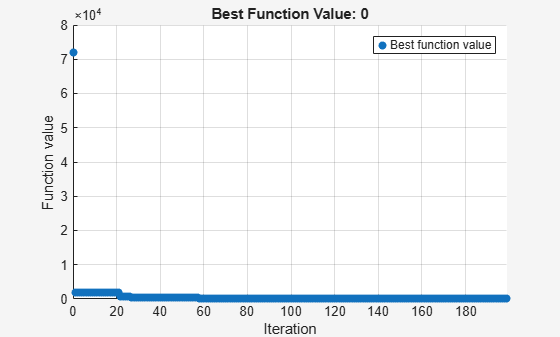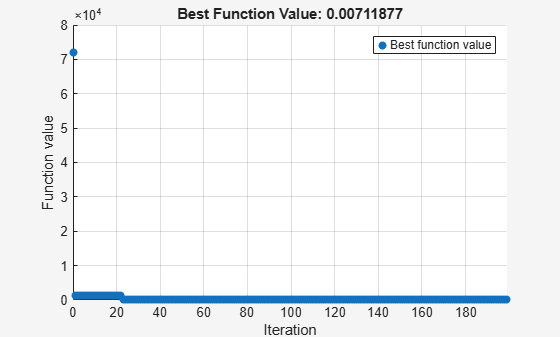# Mixed-Integer Surrogate Optimization, Problem-Based

This example shows how to solve an optimization problem that involves integer variables. In this example, find the point `x` that minimizes the `multirosenbrock` function over integer-valued arguments ranging from –3 to 6 in 10 dimensions. The `multirosenbrock` function is a poorly scaled function that is difficult to optimize. Its minimum value is 0, which is attained at the point `[1,1,...,1]`. The code for the `multirosenbrock` function appears at the end of this example.

Create a 10-D row vector variable `x` of type integer with bounds –3 to 6. When you specify scalar bounds, the bounds apply to all variable components.

`x = optimvar("x",1,10,"LowerBound",-3,"UpperBound",6,"Type","integer");`

To use `multirosenbrock` as the objective function, convert the function to an optimization expression using `fcn2optimexpr`.

`fun = fcn2optimexpr(@multirosenbrock,x);`

Create an optimization problem with the objective function `multirosenbrock`.

`prob = optimproblem("Objective",fun);`

Set the maximum number of function evaluations to 200.

`opts = optimoptions("surrogateopt","MaxFunctionEvaluations",200);`

Solve the problem.

```rng(1,'twister') % For reproducibility [sol,fval] = solve(prob,"Solver","surrogateopt","Options",opts)```
```Solving problem using surrogateopt. ``````surrogateopt stopped because it exceeded the function evaluation limit set by 'options.MaxFunctionEvaluations'. ```
```sol = struct with fields: x: [1 1 1 1 1 1 1 1 1 1] ```
```fval = 0 ```

In this case, `surrogateopt` reaches the correct solution.

### Mixed-Integer Problem

Suppose that only the first six variables are integer-valued. To reformulate the problem, create a 6-D integer variable `xint` and a 4-D continuous variable `xcont`.

```xint = optimvar("xint",1,6,"LowerBound",-3,"UpperBound",6,"Type","integer"); xcont = optimvar("xcont",1,4,"LowerBound",-3,"UpperBound",6);```

Convert `multirosenbrock` to an optimization expression using the input `[xint xcont]`.

`fun2 = fcn2optimexpr(@multirosenbrock,[xint xcont]);`

Create and solve the problem.

```prob2 = optimproblem("Objective",fun2); rng(1,'twister') % For reproducibility [sol2,fval2] = solve(prob2,"Solver","surrogateopt","Options",opts)```
```Solving problem using surrogateopt. ``````surrogateopt stopped because it exceeded the function evaluation limit set by 'options.MaxFunctionEvaluations'. ```
```sol2 = struct with fields: xcont: [1.2133 1.4719 1.1857 1.5003] xint: [1 1 1 1 1 1] ```
```fval2 = 0.9736 ```

This time the integer variables reach the correct solution, and the continuous variables are near the solution, but are not completely accurate.

### Helper Function

This code creates the `multirosenbrock` helper function.

```function F = multirosenbrock(x) % This function is a multidimensional generalization of Rosenbrock's % function. It operates in a vectorized manner, assuming that x is a matrix % whose rows are the individuals. % Copyright 2014 by The MathWorks, Inc. N = size(x,2); % assumes x is a row vector or 2-D matrix if mod(N,2) % if N is odd error('Input rows must have an even number of elements') end odds = 1:2:N-1; evens = 2:2:N; F = zeros(size(x)); F(:,odds) = 1-x(:,odds); F(:,evens) = 10*(x(:,evens)-x(:,odds).^2); F = sum(F.^2,2); end```